1. Innon Knowledge Base
2. Tridium Niagara
3. Java, Program object quick bits

# Analog to Digital converter (IO-DIM4 example)

## How would you convert an analog input signal into 4 digital outputs?

This article is an example which uses the Sontay IO-DIM4 device. The article could be used as a scope to get some insights on the methodology used to play with Java code and tweak it to achieve something similar for other cases/devices.

There is a more straightforward method here - Sontay IO-DIM4 Decoding

#### First, what are we converting?

The conversion is basically between an analog signal (we are using 0-10V in the example below) to 4 digital variables, which are the 4 digital inputs connected to the IO-DIM4.

The conversion table used is taken from the device document as following: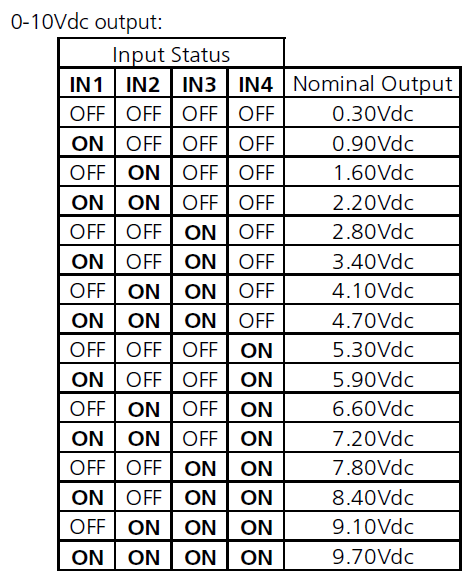Instead of creating an extensive wiresheet with a lot on "MIN" and "MAX" blocks and multiple "OR" blocks to verify when the right conditions are true for each IN1, IN2, IN3 and IN4, I think we can heavily simplify the strategy using JAVA and a few "IF" cycles, with some basic math.

#### Desired outcome

I would like to get 1 block where I can feed my analog signal and get the 4 digital converted variables as outputs.

I would like also to be able to adjust the minimum and maximum value (maybe I want to use 0-100% instead of 0-10V or 4-20mA perhaps?)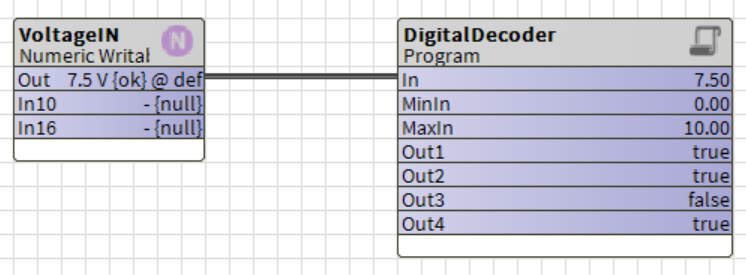Note: if you have doubts on the next steps and how to use a Program object, THIS BASIC ARTICLE might be useful, to start simple.

#### The slots

I will need 3 input slots (my signal and minimum/maximum value in) and 4 output slots (the boolean converted outputs).

Once in the "Program Editor" view, and using the "Slots" tab, I added the 3 inputs selected as "Baja - Double" and flagged as "Execute on change" and "Summary"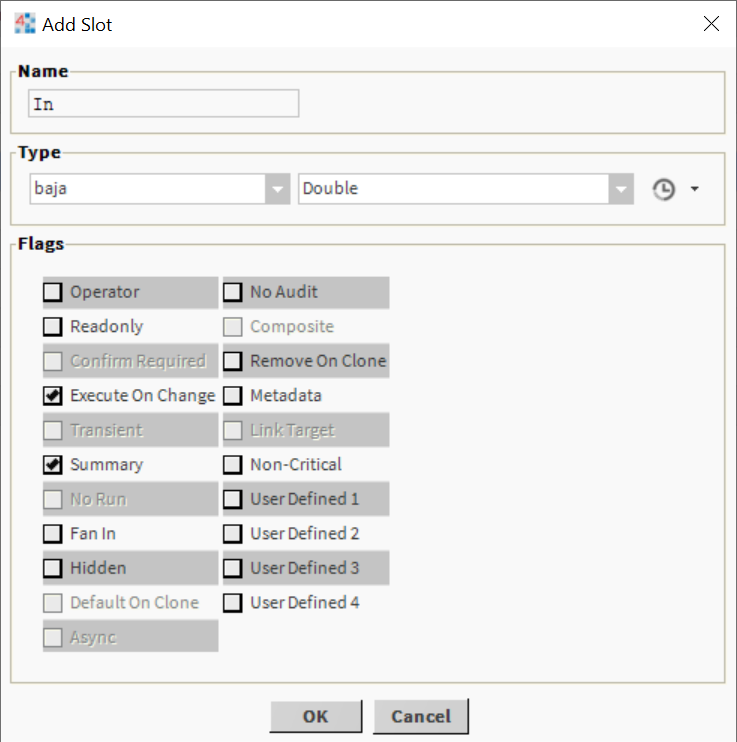And then the 4 outputs as "Baja - Boolean" and flagged as "Readonly" and "Summary"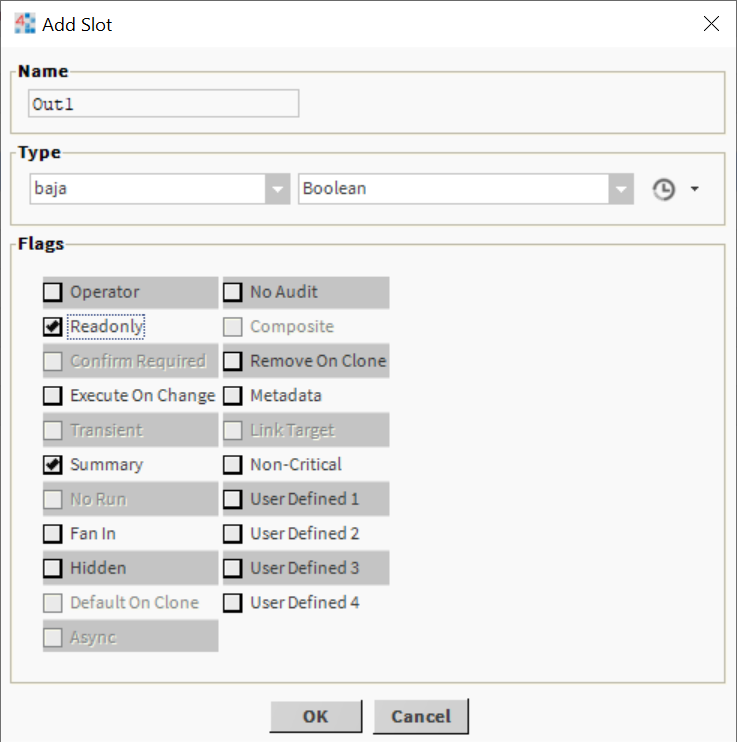Here is how they should look like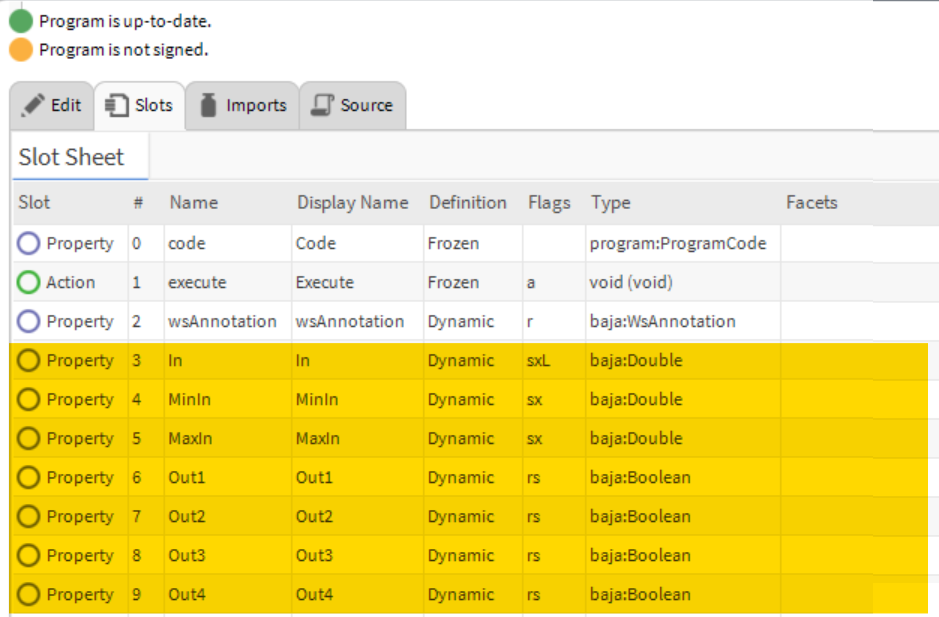#### The code

You should be able to read the code through the lines, but I have added a few comments to clarify what the code does.

In essence, it identifies the steps first, and then for each boolean output it goes through each case ("FOR" cycle) and verifies if there is a condition met to set it to "true".

Because the steps are equally distributed between 0 and 10V input (or minimum and maximum input to be precise), then the verification can be achieved this way. If the steps were not equally distributed, we would probably need to find a different method

`public void onStart() throws Exception{  // start up code here}public void onExecute() throws Exception{  // execute code (set executeOnChange flag on inputs)    // Get the inputs to auxiliary variables  double maxInput = getMaxIn();  double minInput = getMinIn();  double currInput = getIn();    // declare and reset the auxiliary variables for the outputs  boolean outBool1 = false;  boolean outBool2 = false;  boolean outBool3 = false;  boolean outBool4 = false;    // Prepare the steps for each boolean output calculation  double steps16 = (maxInput - minInput) / 16;  double steps8 = (maxInput - minInput) / 8;  double steps4 = (maxInput - minInput) /4;  double steps2 = (maxInput - minInput) /2;    // Filter inputs above maximum and below minimum  if (currInput < minInput) {     currInput = minInput;  }    if (currInput > maxInput) {     currInput = maxInput;  }       // Calculate output 1  for (double i = (minInput + steps16); i <= maxInput; i = i + (steps16 * 2)) {     if (currInput > i && currInput <= (i + steps16)) {     outBool1 = true;     }  }    // Calculate output 2  for (double i = (minInput + steps8); i <= maxInput; i = i + (steps8 * 2)) {     if (currInput > i && currInput <= (i + steps8)) {     outBool2 = true;     }  }  // Calculate output 3  for (double i = (minInput + steps4); i <= maxInput; i = i + (steps4 * 2)) {     if (currInput > i && currInput <= (i + steps4)) {     outBool3 = true;     }  }  // Calculate output 4  if (currInput > (minInput + steps2)) {     outBool4 = true;  }  // Write all the outputs  setOut1(outBool1);  setOut2(outBool2);  setOut3(outBool3);  setOut4(outBool4);  }public void onStop() throws Exception{  // shutdown code here}`

Once you compile your Program Object, you should see the block as displayed in this article "Desired outcome" and it should work as intended.

If you need to sign your Program object, we have an article on how to sign it HERE